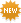Let The Message Run Free lyrics by Boyzone - original song full text. Official Let The Message Run Free lyrics, 2019 version | LyricsMode.comRequest & respond explanations
• Don't understand the meaning of the song?
• Highlight lyrics and request an explanation.
• Click on highlighted lyrics to explain.
Boyzone – Let The Message Run Free lyrics
In this world of confusion
Under the same sun
Save us from complication
Let the message run
I was speaking so many tongues
In a voice loud and clear
We'll bridge communications
Let the message run

So do I have to talk to another?
Can we speak as one?
And if we talk to each other
We should all be
We should all be
We should all be one
One

Let us run free
Oh let us see (oh let us see)
And through the eyes of a child
Let the message run free (free)

Let us run free (let us run free)
Oh let us see (oh let us see)
Through the eyes of a child
Let the message run free
(let the message run, let the message run)

Through the wires like lightening
Over land and ocean, yeah
No more in isolation
We should all be
We should all be
We should all be one
One

Let us run free (let us run free)
Oh let us see (oh let us see)
Through the eyes of a child
Let the message run free
(let the message run, let the message run)

(let's run free...)
Let us run free
(oh, let us run free)
Oh let us see
(oh let us see)
Through the eyes of a child
Let the message run free
(let the message run, let the message run free)

Through the wires like lightening
Over land and ocean
No more in isolation
Let the message run free
Let the message run free

Let us run free
(let us run free)
Oh let us see
(oh let us see)
Through the eyes of a child
Let the message run free
×

Lyrics taken from /lyrics/b/boyzone/let_the_message_run_free.html

• Email
• Correct

Let The Message Run Freemeanings

Write about your feelings and thoughts about Let The Message Run Free

Know what this song is about? Does it mean anything special hidden between the lines to you? Share your meaning with community, make it interesting and valuable. Make sure you've read our simple .
U
Min 50 words
Good
Awesome!

U
Min 50 words